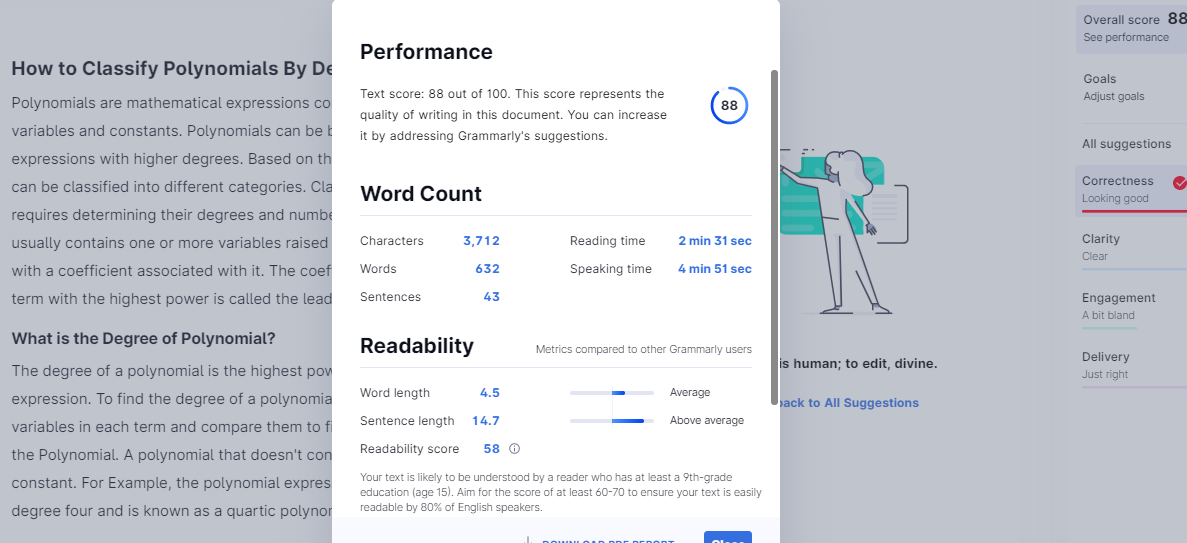# How to Classify Polynomials By Degree?

on May 4, 2021

Polynomials are mathematical expressions consisting of terms comprising variables and constants. Polynomials can be both simple and complex expressions with higher degrees. Based on the degree of a polynomial, it can be classified into different categories. Classifying the polynomials requires determining their degrees and number of terms. Each term usually contains one or more variables raised to some exponential powers with a coefficient associated with it. The coefficient associated with the term with the highest power is called the leading coefficient.

## What is the Degree of Polynomial?

The degree of a polynomial is the highest power of an algebraic expression. To find the degree of a polynomial, add the powers of variables in each term and compare them to find the largest exponent in the polynomial. A polynomial that doesn’t contain any variable is called a constant. For example, the polynomial expression 2x²y² + 4x²y + 9 has degree four and is known as a quartic polynomial.

## Types of Polynomials Based on the Degree:

The highest value of the exponent in an expression is known as the degree of polynomial or order of the polynomial. While finding the degree of the polynomial, the terms should be arranged in either ascending or descending order. Based on the degree of the polynomial, polynomials can be classified into four major types:

### Zero or Constant Polynomial:

A constant polynomial is a polynomial without a variable which means it has only a constant part. For example, 2, -5, etc. Since there is no variable in such polynomials, we can say it is a polynomial with zero degrees.

### Linear Polynomial:

The polynomial expression whose degree is one is called a linear polynomial—for Example, 2x+7 or 6y+3.

The polynomial expression with the highest degree as two is called a quadratic polynomial. For example 2x² + 5 or 16y² + 7.

### Cubic Polynomial:

The polynomial expression whose degree is three is called a cubic polynomial. For example, 7x²y + 5xy + 8.

### Quartic Polynomials:

The polynomial expression whose degree is four is called a quartic polynomial. For example, 8x²y² + 3x² + 2y.

## How to find the Degree of Polynomial?

Following are simple steps to find the degree of a polynomial in a variety of situations:

### Degree of a Polynomial with one Variable:

Finding the degree of a polynomial with one variable requires arranging the exponents in decreasing order, with the highest value in the first place and the lowest value at last. For example, in this expression -x^5 + x^4 + x, the first term has power 5. The power of a polynomial is only the number in the exponent. Since you’ve arranged the polynomial to put the largest exponent first, that will help you to find the largest term, which is the degree of this polynomial.

### Degree of a Constant Polynomial:

The degree of a constant is zero. If the polynomial contains only a constant value such as 33 or 56, then the degree of this type of polynomial is zero. You can also consider this polynomial as the constant term attached to a variable with the power of 0, which is 1. For example, consider the polynomial 12; you can think of it as 15x^0, 12 x 1, or 12; Which implies that the degree of a constant polynomial is 0.

### Degree of a Polynomial with Multiple Variables

Finding a polynomial degree with multiple variables is quite trickier than finding the degree of a polynomial with constants and one variable. To find the degree of a polynomial with multiple degrees, simply add the powers of the variables in each term; it is not required that they are the same variables. The degree of a variable without any degree, such as x or y, is just one.